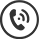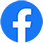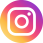Call Us 03 349 7862

# EQUIPMENT TIPS

## How To Choose A Pump Size

1 - First decide the maximum flow rate you will require using the following formula:
2 - Boom width: e.g. 12 metre
3 - Maximum travel speed: e.g. 7 kph
4 - Application rate: e.g. 200 litres per hectare.
5 - =12mx 7kph x 200 lit/ha   = 16800

Constant factor               600       =     28 litres per min for boom

6 - Calculate 5% of the volume of your tank capacity for agitation:

e.g. 1000 litre tank = 50 litres

7 - Add the two answers: e.g. 28 +50 = Minimum pump size = 78 litres per min.

A hand gun sprayer normally requires up to12 litres per minute per gun.

## Sprayer Calibration

### Speed Check

Knowing your real tractor speed is an essential part of accurate spraying. Speedometer readings can be inaccurate because of wheel slippage. The most accurate method is to half fill your sprayer tank with water the measure out a suitable distance in a field with a tape measure. e.g.100 metres.

Select a suitable gear and time your vehicle over this measured distance.

The formula  to calculate your speed is:-

Distance in metres multiplied by 3.6 and divide this figure by the time in seconds taken to cover the distance.

Example:  100 (metres)  x   3.6        360

36 (seconds)   = 10 kph

### Nozzle Size Calculator

To determine the flow rate per nozzle required, the following formula is used:

The desired litre per ha rate is multiplied by speed in kph and this figure is multiplied by the nozzle spacing in cm. The figure is divided by 60,000

Example:  200 (Lit/ha) x 10 (kph) x 50 (cm)      =    100,000

60,000   = 1.66 lit/min

Go to a nozzle chart and select a nozzle that will give an application rate of 1.66 lit/min at between 2 and 3 bar.  A Teejet XR11005 will give this rate at about 2.2 bar.

Adjust the pressure until a catch test of a number of nozzles gives  the correct flow rate.

 Phone: 03 349 7862 Fax: 03 349 7641PARTS: parts@nobleadams.co.nz SALES:aaron@nobleadams.co.nz WORKSHOP: barry@nobleadams.co.nz 66 Waterloo Road Hornby Christchurch New Zealand